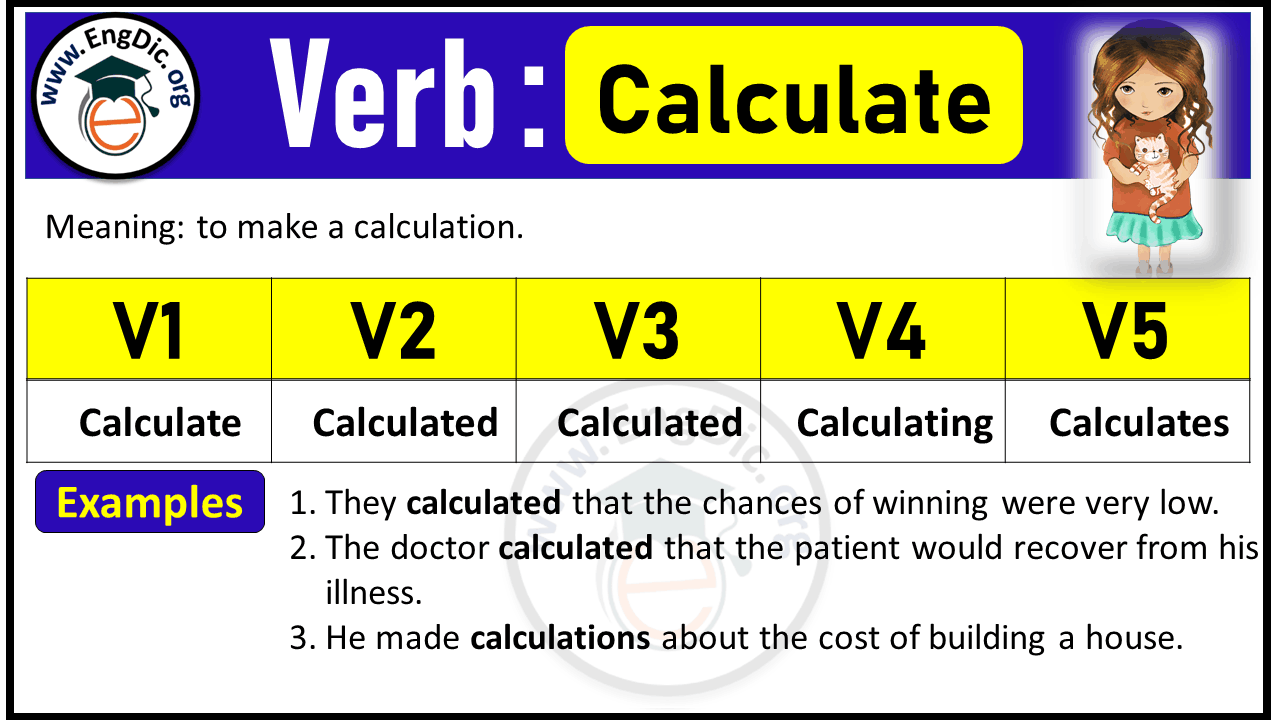# Calculate Verb Forms: Past Tense and Past Participle (V1 V2 V3)

Meaning: to make a calculation.

## Calculate Verb Forms V1 V2 V3 V4 V5

 Infinitive/Base Form (V1) Calculate Past Tense (V2) Calculated Past Participle Form (V3) Calculated Present Participle/Gerund (V4) Calculating 3rd Person Singular (V5) Calculates

## Calculate Past Tense:

Past Tense of Calculate is Calculated.

Example: Sarah calculated the expenses.

## Calculate Past Participle:

Past Participle Form of Calculate is Calculated.

Example: Sarah has calculated the expenses.

## Calculate Present Participle:

Present Participle Form of Calculate is Calculating.

Example: Sarah is calculating the expenses.

## Calculate 3rd Person Singular:

3rd Person Singular of Calculate is Calculates.

Example: Sarah calculates the expenses.## Calculate Conjugation

### Indefinite / Simple Present Tense

• I calculate the expenses.
• We/You/They calculate the expenses.

### Present Continuous Tense

• I am calculating the expenses.
• We/You/They are calculating the expenses.
• He/She/It/Adam is calculating the expenses.

### Present Perfect Tense

• I have calculated the expenses.
• We/You/They have calculated the expenses.
• He/She/It/Adam has calculated the expenses.

### Present Perfect Continuous Tense

• I have been calculating the expenses.
• We/You/They have been calculating the expenses.
• He/She/It/Adam has been calculating the expenses.

### Indefinite / Simple Past Tense

• I calculated the expenses.
• We/You/They calculated the expenses.

### Past Continuous Tense

• I was calculating the expenses.
• We/You/They were calculating the expenses.
• He/She/It/Adam was calculating the expenses.

### Past Perfect Tense

• I had calculated the expenses.
• We/You/They had calculated the expenses.

### Past Perfect Continuous Tense

• I had been calculating the expenses.
• We/You/They had been calculating the expenses.

### Indefinite / Simple Future Tense

• I will calculate the expenses.
• We/You/They will calculate the expenses.
• He/She/It/Adam will calculate the expenses.

### Future Continuous Tense

• I will be calculating the expenses.
• We/You/They will be calculating the expenses.
• He/She/It/Adam will be calculating the expenses.

### Future Perfect Tense

• I will have calculated the expenses.
• We/You/They will have calculated the expenses.
• He/She/It/Adam will have calculated the expenses.

### Future Perfect Continuous Tense

• I will have been calculating the expenses.
• We/You/They will have been calculating the expenses.
• He/She/It/Adam will have been calculating the expenses.

## Past Tense of Calculate Phrasal Verbs

 Calculate Phrasal Verbs Past Tense Calculate out Calculated out Calculate up Calculated up Calculate for Calculated for Calculate on Calculated on Calculate in Calculated in Calculate through Calculated through Calculate with Calculated with Calculate over Calculated over Calculate back Calculated back Calculate off Calculated off

## FAQS

### What is the Future Tense of Calculate?

Future Tense of calculate is “will calculate”.

### What is the Present Tense of Calculate?

Present Tense of calculate is “calculate + s/es or ing”.

 I/we/you/they calculate He/she/it/singular name calculates Present participle calculating

### What is the Past Perfect Tense of Calculate?

Past perfect tense of take is “had calculated”.

Explore Other Verb Forms:

Last updated on May 22nd, 2023 at 04:01 pm